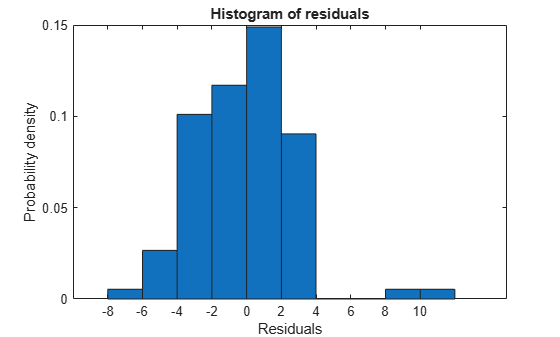Documentation

### This is machine translation

Mouseover text to see original. Click the button below to return to the English version of the page.

To view all translated materials including this page, select Country from the country navigator on the bottom of this page.

# plotResiduals

Class: LinearModel

Plot residuals of linear regression model

## Syntax

```plotResiduals(mdl) plotResiduals(mdl,plottype) h = plotResiduals(___) h = plotResiduals(mdl,plottype,Name,Value) ```

## Description

`plotResiduals(mdl)` gives a histogram plot of the residuals of the `mdl` linear model.

`plotResiduals(mdl,plottype)` plots residuals in a plot of type `plottype`.

`h = plotResiduals(___)` returns handles to the lines in the plot, using any of the previous syntaxes.

`h = plotResiduals(mdl,plottype,Name,Value)` plots with additional options specified by one or more `Name,Value` pair arguments.

## Input Arguments

expand all

Full, fitted linear regression model, specified as a `LinearModel` object constructed using `fitlm` or `stepwiselm`.

Plot type, specified as one of the following:

 `'caseorder'` Residuals vs. case (row) order `'fitted'` Residuals vs. fitted values `'histogram'` Histogram `'lagged'` Residuals vs. lagged residual (r(t) vs. r(t–1)) `'probability'` Normal probability plot `'symmetry'` Symmetry plot

### Name-Value Pair Arguments

Specify optional comma-separated pairs of `Name,Value` arguments. `Name` is the argument name and `Value` is the corresponding value. `Name` must appear inside quotes. You can specify several name and value pair arguments in any order as `Name1,Value1,...,NameN,ValueN`.

### Note

The plot property name-value pairs apply to the first returned handle `h(1)`.

Color of the line or marker, specified as the comma-separated pair consisting of `'Color'` and a `ColorSpec` specification. For details, see `linespec`.

Line style, specified as the comma-separated pair consisting of `'LineStyle'` and a Line Properties specification. For details, see `linespec`.

Width of the line or edges of filled area, in points, specified as the comma-separated pair consisting of `'LineWidth'` and a positive numeric value. One point is equal to 1/72 inch.

Color of the marker or edge color for filled markers, specified as the comma-separated pair consisting of `'MarkerEdgeColor'` and a `ColorSpec` specification. For details, see `linespec`.

Color of the marker face for filled markers, specified as the comma-separated pair consisting of `'MarkerFaceColor'` and a `ColorSpec` specification. For details, see `linespec`.

Size of the marker in points, specified as the comma-separated pair consisting of `'MarkerSize'` and a positive numeric value. One point is 1/72 inch.

Type of residual used in the plot, specified as the comma-separated pair consisting of `'ResidualType'` and one of the following:

 `'raw'` Observed minus fitted values `'pearson'` Raw residuals divided by RMSE `'standardized'` Raw residuals divided by their estimated standard deviation `'studentized'` Raw residuals divided by an independent (delete-1) estimate of their standard deviation

## Output Arguments

expand all

Graphics handles, returned as a vector of graphics handles corresponding to the lines or patches in the plot.

## Examples

expand all

Plot a histogram of the residuals of a fitted linear model.

Load the `carsmall` data and fit a linear model of the mileage as a function of model year, weight, and weight squared.

```load carsmall tbl = table(MPG,Weight); tbl.Year = ordinal(Model_Year); mdl = fitlm(tbl,'MPG ~ Year + Weight^2');```

Plot the raw residuals.

`plotResiduals(mdl)`Create a normal probability plot of the residuals of a fitted linear model.

Load the `carsmall` data and fit a linear model of the mileage as a function of model year, weight, and weight squared.

```load carsmall X = [Weight,Model_Year]; mdl = fitlm(X,MPG,... 'y ~ x2 + x1^2','Categorical',2);```

Create a normal probability plot of the residuals of the fitted model.

`plotResiduals(mdl,'probability')`## Tips

• For many plots, the Data Cursor tool in the figure window displays the x and y values for any data point, along with the observation name or number.

## Alternatives

The `mdl.Residuals` table contains the information in residual plots.